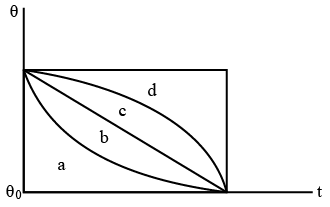# ISRO Scientist or Engineer Mechanical 2014

Instructions

For the following questions answer them individually

Question 1

# Shock resisting steel should have higherQuestion 2

# Let $$A = \begin{bmatrix}0 & 0 & -1 \\0 & -1 & 0 \\-1 & 0 & 0 \end{bmatrix}$$ The only correct statement about the matrix A isQuestion 3

# Let M be a $$3 \times 3$$ matrix satisfying:$$M \begin{bmatrix}0\\1\\0 \end{bmatrix} = \begin{bmatrix}-1\\2\\3 \end{bmatrix}, M \begin{bmatrix}1\\-1\\0 \end{bmatrix} = \begin{bmatrix}1\\1\\-1 \end{bmatrix}$$, and $$M \begin{bmatrix}1 \\1 \\1 \end{bmatrix} = \begin{bmatrix}0 \\0 \\12 \end{bmatrix}$$. Then the sum of the diagonal entries of M is:Question 4

# Angle made between vector $$\overrightarrow{C} = \overrightarrow{2i} - \overrightarrow{3j} + \overrightarrow{4k}$$ and the Z-axisQuestion 5

# Which of the following is the correct definition of the Reynolds number?Where $$\rho$$ = fluid density, U = velocity, L = Length and $$\mu$$ = kinematic viscosityQuestion 6

# A steam engine intakes 100gm of steam at $$100^\circ C$$ per minute and cools it down to $$20^\circ C$$ . Calculate the heat rejected by the steam engine per minute. (consider Latent Heat of vaporization of steam = 540 cal/gm and Cp = 1 cal/gm/$$^\circ C$$Question 7

# A heat engine operates between a cold reservoir at temperature T2 = 300 K and a hot reservoir at Temperature T1. It takes 200J of heat from the hot reservoir and deliver 120J of heat to the cold reservoir in a cycle. Whatwill be the efficiency of the engine?Question 8

# Two bodies A and B having equal surface area are maintained at temperatures $$10^\circ C$$ and $$20^\circ C$$. The energy of thermal radiations emitted in a given time by A and B are in the ratio:Question 9

# A hot liquid is kept in a big room. Its temperature($$\theta$$) is plotted as a function of time(t), which of the following curve may represent the plot?Question 10

# Aluminum crystal structure isOR